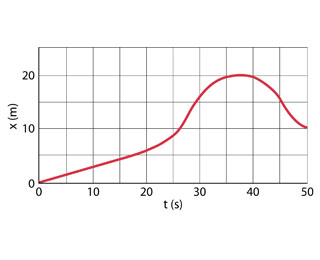# Problem: The position of a rabbit along a straight tunnel as a function of time is plotted in the figure.a.) What is its average velocity between t = 0 and t = 5.0s?b.) What is its average velocity between t = 25.0s and t = 30.0s?c.) What is its average velocity between t = 40.0s and t = 50.0s?

###### FREE Expert Solution

Average velocity:

a)

From the graph, the displacement from 0 s to 5.0 s is approximately 1.5 m.

85% (201 ratings)###### Problem Details

The position of a rabbit along a straight tunnel as a function of time is plotted in the figure.a.) What is its average velocity between = 0 and = 5.0s?

b.) What is its average velocity between = 25.0s and = 30.0s?

c.) What is its average velocity between = 40.0s and = 50.0s?